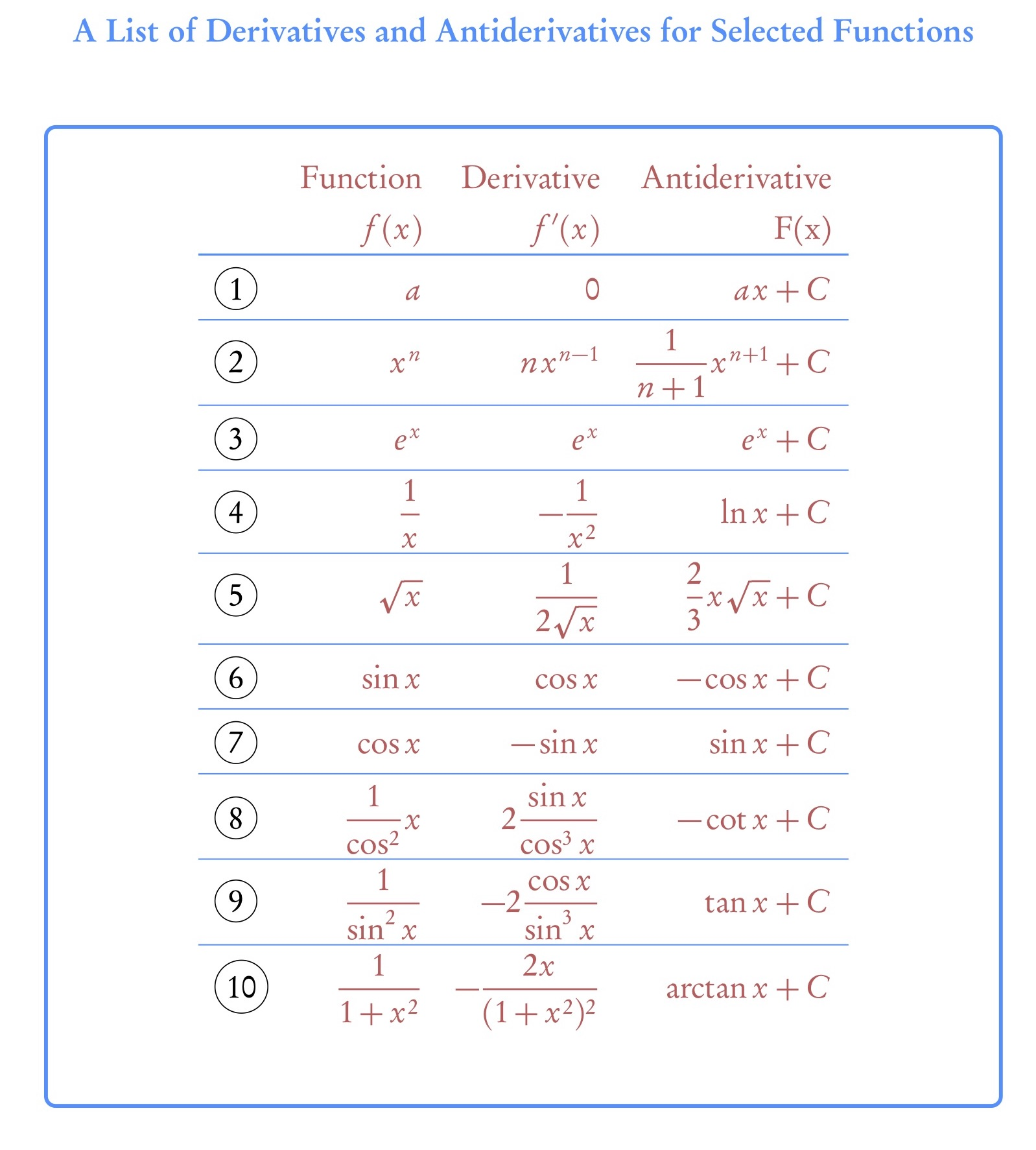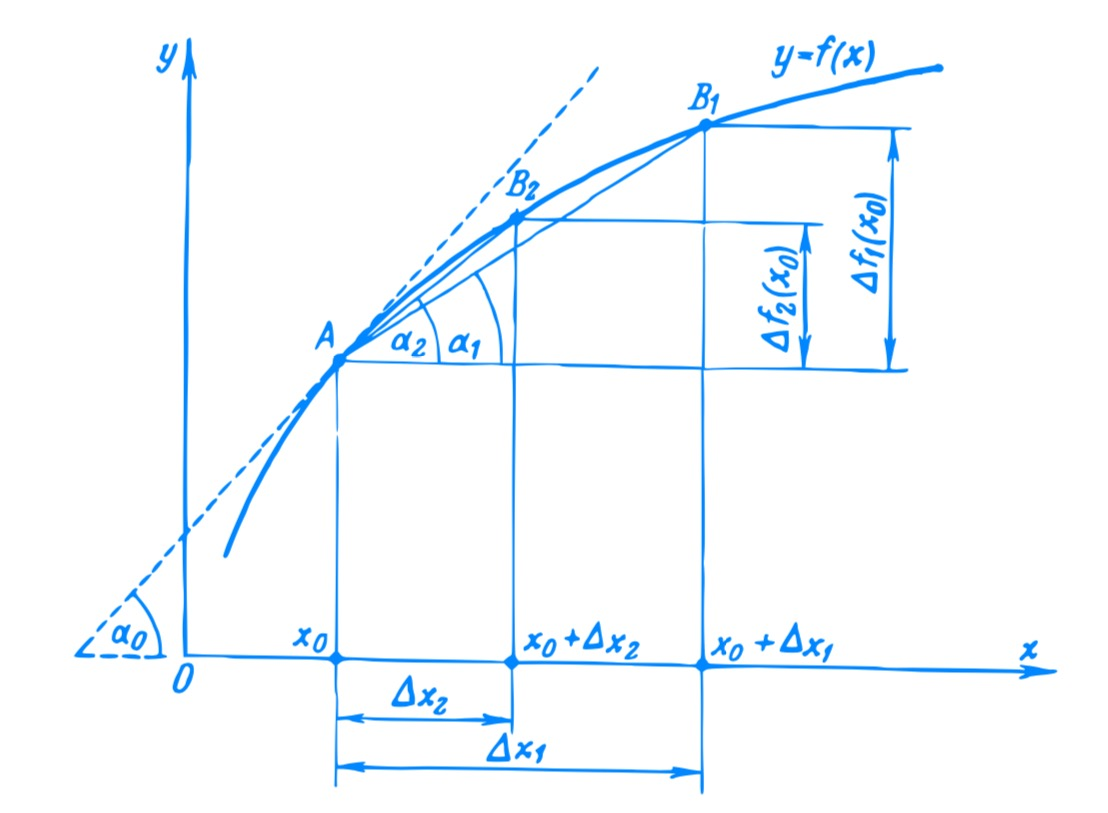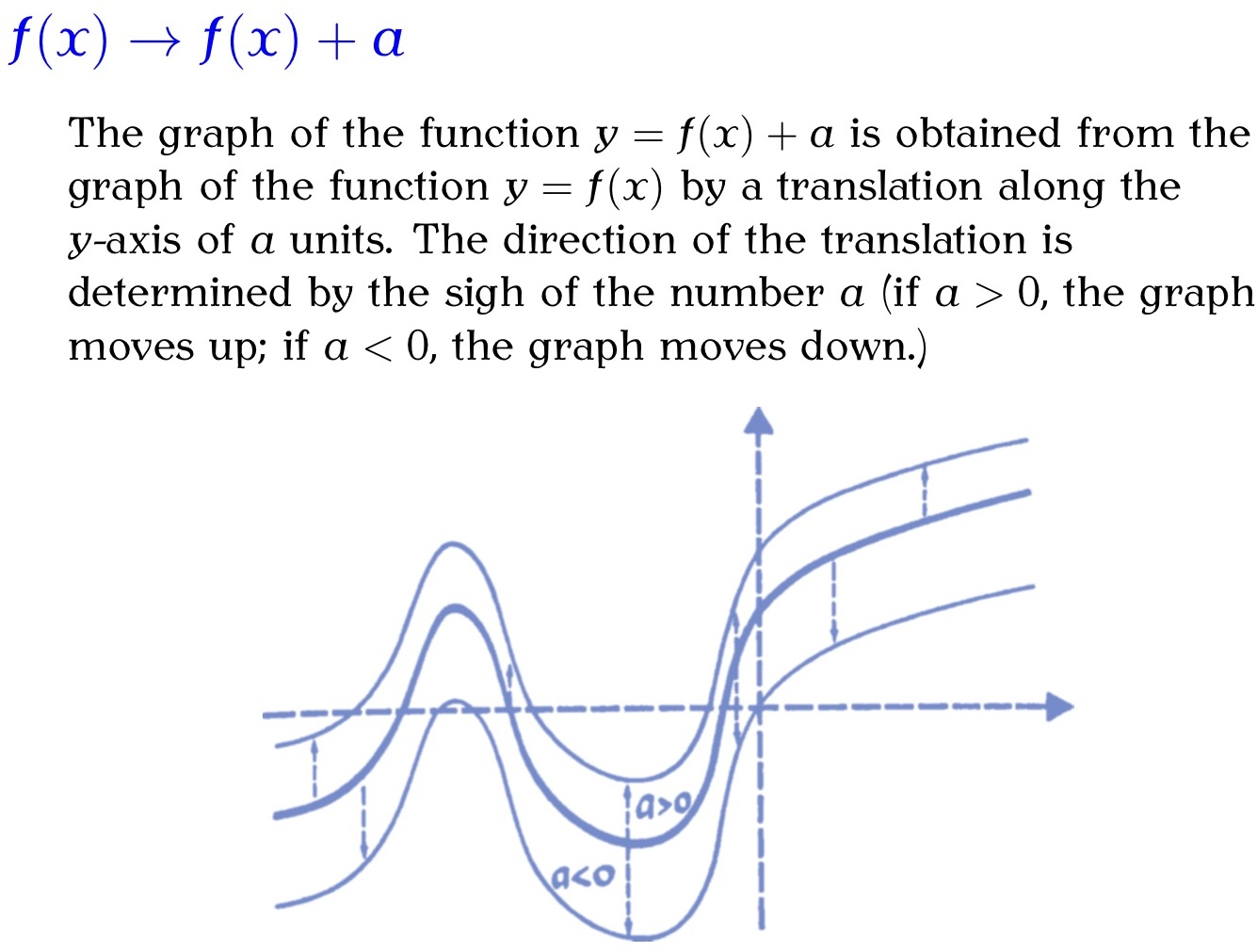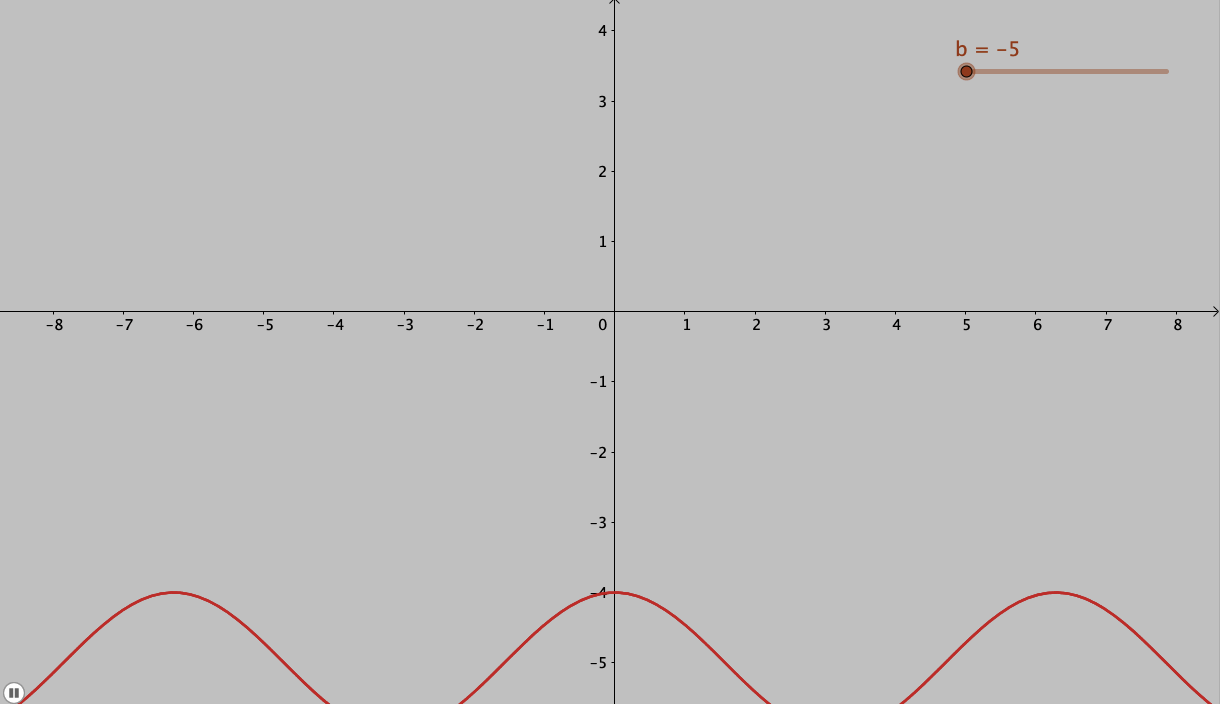# What is the mathematical significance of the constant C in an indefinite integral?

As we had seen in an earlier post, calculus bottleneck, calculus presents one of the most difficult topics for the students in higher mathematics. But the problem is not just limited to the students. Teachers feel it too. Too often the emphasis is given on how to solve integration and differentiation problems using “rules” and “methods” while the essence of what is happening is lost. Recently, I asked this question in an interview to a mathematics teacher who was teaching indefinite integration. This teacher had almost a decade of experience in teaching mathematics at +2 level. The teacher tried to answer this question by using an example of the function $$x^{2} + 5$$. Now when we take the derivative of this function, we get

$\dv{ (x^{2} + 5)}{x} = \dv{x^{2}}{x} + \dv{5}{x} = 2x$

as derivative of a constant (5 on our case) is 0). Now the teacher tried to argue, that integration is the reverse of the derivative), so

$\int 2x \, \dd x = \frac{2x^{2}}{2} + C = 2x + C$

After this the teacher tried to argue this $$C$$ represents the constant term (5) in our function $$x^{2} + 5$$. He tried to generalise the result, but he was thinking concretely in terms of the constant in the form of the numbers in the function. The teacher could understand the mechanism of solving the problem, but was not able to explain in clear mathematical terms, why the constant $$C$$ was required in the output of the indefinite integral. This difficulty, I think, partly arose because the teacher only thought in terms solving integrals and derivatives in a particular way, and knew about the connection between the two, but not in a deep way. He did in a way understood the essence of the constant $$C$$, but was not able to understand my question as a general question and hence replied only in terms of concrete functions. Even after repeated probing, the teacher could not get the essence of the question:

why do we add a constant term to the result of the indefinite integral?

To put it in another words, he was not able to generalise an abstract level of understanding from the examples that were discussed. The teacher was thinking only in terms of symbol manipulation rules which are sufficient for problem solving of these types. For example, look at the corresponding rules for differentiation and integration of the function $$x^{n}$$.

$\dv{x^{n}}{x} = n x^{n-1} \iff \int x^{n} \dd x = \frac{x^{n+1}}{n} + C$Thus, we see according to above correspondence that adding any extra constant $$C$$ to the derivative formula will not affect it. So the teacher claimed it is this constant which appears in the integration rule as well. In a way this is a sort of correct explanation, but it does not get to the mathematical gist of why it is so. What is the core mathematical idea that this constant $$C$$ represents.

Another issue, I think, was the lack of any geometrical interpretation during the discussion. If you ask, what is the geometrical interpretation of the derivative you will get a generic answer along the lines: “It is the tangent to the curve” and for integration the generic answer is along the lines “It is the area under the curve”. Both these answers are correct, but how do these connect to the equivalence above? What is the relationship between the tangent to the curve and area under the curve which allows us to call the integral as the anti-derivative (or is the derivative an anti-integral?). I think to understand these concepts better we have to use the geometrical interpretation of the derivative and the integral from their first definitions.

The basic idea behind the derivative is that it represents the rate of change of a function $$f$$ at a given point. This idea, for an arbitrary function, can be defined (or interpreted) geometrically as:

The derivative of a function $$f$$ at a point $$x_{0}$$ is defined by the slope of the tangent to the graph of the function $$f$$ at the point  $$x = x_{0}$$.

The animation below shows how the slope of the tangent to the sine curve changes. Point $$B$$ in the animation below plots the $$(x, m)$$, where $$m$$ is the slope of the tangent for the given value of $$x$$. Can you mentally trace the locus of point $$B$$? What curve is it tracing?Now, the tangent to any point on a curve is unique. (Why is it so?) That means if one evaluates a derivative of a function at a point, it will be a unique result for that point.This being cleared, now let us turn to the indefinite integral. One approach to understanding integration is to consider it as an inverse operation to the derivative, i.e. an anti-derivative.

An anti-derivative is defined as a function $$F(x)$$ whose derivative equals an initial function $$f (x)$$:

$f(x)= \dv{ F(x)}{x}$

Let us take an example of the function $$f(x) = 2x^{2} – 3x$$. The differentiation of this function gives us its derivative $$f'(x) = 4x – 3$$, and its integration gives us anti-derivative.
$F(x) = \frac{2}{3} x^{3} – \frac{3}{2} x^2$

This anti-derivative can be obtained by applying the known rules of differentiation in the reverse order. We can verify that the differentiation of the anti-derivative leads us to the original function.

$F'(x) = \frac{2}{3} 3 x^{2} – \frac{3}{2} 2 x = 2x^{2} – 3x$

Now if add a constant to the function $$F(x)$$, lets say number 4, we get a function $$G(x) = \frac{2}{3} x^{3} – \frac{3}{2} x^2 + 4$$. If we take the derivative of this function $$G(x)$$, we still get our original function back. This is due to the fact that the derivative of a constant is zero. Thus, there can be any arbitrary constant added to the function $$F(x)$$ and it will still be the anti-derivative of the original function $$f(x)$$.

An anti-derivative found for a given function is not unique. If $$F (x)$$ is an anti-derivative (for a function $$f$$ ), then any function $$F(x)+C$$, where $$C$$ is an arbitrary constant, is also an anti-derivative for the initial function because
$\dv{[F(x)+C]}{x} = \dv{ F(x)}{x} + \dv{ C}{x}= \dv{ F(x) }$

But what is the meaning of this constant $$C$$? This means, that each given function $$f (x)$$ corresponds to a family of anti-derivatives, $$F (x) + C$$. The result of adding a constant $$C$$ to any function is that it shifts along the $$Y$$-axis.Thus what it means for our case of result of the anti-derivative, the resultant would be a family of functions which are separated by $$C$$. For example, let us look at the anti-derivative of $$f (x) = \sin x$$. The curves of anti-derivatives for this function are plotted in will be of the form

$F ( x ) = − \cos x + C$A family of curves of the anti-derivatives of the function $$f (x) = \sin x = – \cos x$$

And this is the reason for adding the arbitrary constant $$C$$ to our result of the anti-derivative: we get a family of curves and the solution is not unique.

Now can we ever know the value of $$C$$? Of course we can, but for this we need to know the some other information about the problem at hand. These can be initial conditions (values) of the variables or the boundary condition. Once we know these we can determine a particular curve (particular solution) from the family of curves for that given problem.

Lev Tarasov – Calculus – Basic Concepts For High Schools (Starts with and explains  the basic mathematical concepts required to understand calculus. The book is in the form of a dialogue between the author and the student, where doubts, misconceptions and aha moments are discussed.)

Morris Kline – Calculus – A physical and intuitive approach (Builds the concepts in the context of the physical problems that calculus was invented to solve.  A book every physics student should read to get an understanding of how mathematics helps solve physical problems.)

Richard  Courant and Fritz John – Introduction to Calculus Analysis (In 2 Volumes) (Standard college level text with in-depth discussions. First volume is rigorous with basic concepts required to conceptually understand the topics and their applications/implications.)

# John Tukey on data based pictures and graphs

John Tukey‘s wisdom on importance and value of graphics and pictures in making sense of exploring data.

Consistent with this view, we believe, is a clear demand that pictures based on exploration of data should force their messages upon us. Pictures that emphasize what we already know — “security blankets” to reassure us — are frequently not worth the space they take. Pictures that have to be gone over with a reading glass to see the main point are wasteful of time and inadequate of effect. The greatest value of a picture is when it forces us to notice what we never expected to see. (p. vi emphasis in original)

John Tukey – Exploratory Data Analysis

# The True Purpose Of Graphic Display – J. W. Tukey

John Wilder Tukey, one of the greatest Statistician of the last century points to what the purpose of a graphic display should be:

1.  Graphics are for the qualitative/descriptive – conceivably the semi quantitative – never for the carefully quantitative (tables do that better).
2. Graphics are for comparison – comparison of one kind or another – not for access to individual amounts.
3. Graphics are for impact – interocular impact if possible, swinging-finger impact if that is the best one can do, or impact for the unexpected as a minimum – but almost never for something that has to be worked at hard to be perceived.
4. Finally, graphics should report the results of careful data analysis – rather than be an attempt to replace it. (Exploration-to guide data analysis – can make essential interim use of graphics, but unless we are describing the exploration process rather than its results, the final graphic should build on the data analysis rather than the reverse.)

From:

Tukey, J. W. (1993). Graphic comparisons of several linked aspects: Alternatives and suggested principles. Journal of Computational and Graphical Statistics, 2(1), 1-33.